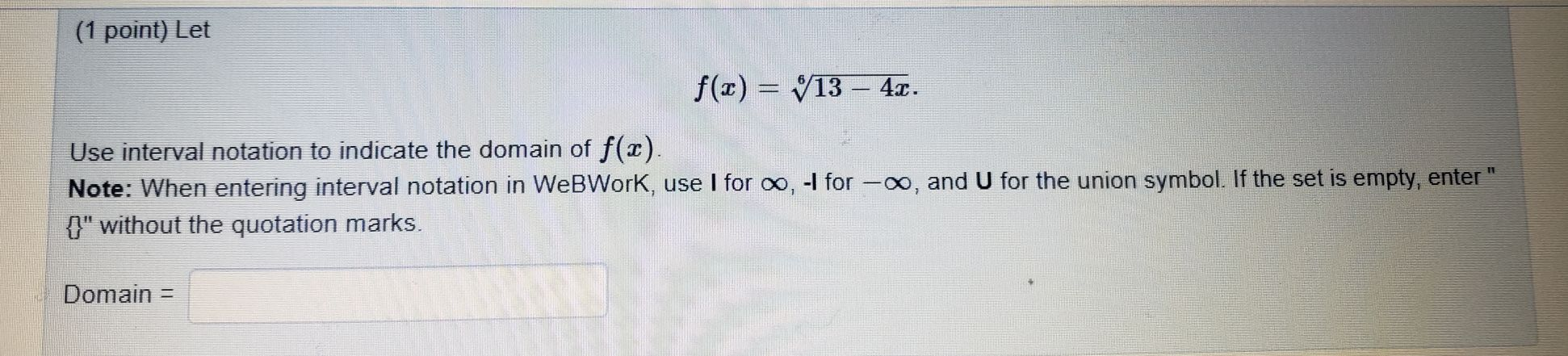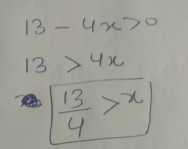### ¿Todavía tienes preguntas de matemáticas?

Pregunte a nuestros tutores expertos
Algebra
Pregunta(1 point) Let $$f ( x ) = \sqrt[ 6 ] { 13 - 4 x }$$

Use interval notation to indicate the domain of $$f ( x )$$ . Note: When entering interval notation in WeBWorK, use I for $$\infty , - I$$ for $$- \infty$$ , and $$U$$ for the union symbol. If the set is empty, enter "

$$\{\} ^ { \prime \prime }$$ without the quotation marks.

Domain =